# Debt

Joe and Caryl have a debt of \$100,500. Joe makes \$90,000 per year, and Caryl makes \$35,000 per year. How much should both pay to zero out the debt fairly, based on their salaries?

Correct result:

c =  28140
j =  72360

#### Solution:

j+c = 100500
j = 90000/35000•c

c+j = 100500
90000c-35000j = 0

c = 28140
j = 72360

Our linear equations calculator calculates it.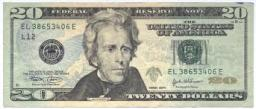We would be pleased if you find an error in the word problem, spelling mistakes, or inaccuracies and send it to us. Thank you!Tips to related online calculators
Check out our ratio calculator.
Do you have a linear equation or system of equations and looking for its solution? Or do you have quadratic equation?

## Next similar math problems:

• 120 nuts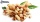Divide 120 nuts in a ratio of 4: 6.
• Proportional relationship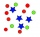The ordered pairs (6,24) and (1, s) represent a proportional relationship. Find the value of s.
• Two xeroxes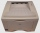The performances of the two copiers are in the ratio 3: 4. A machine with higher power will make 7,200 copies in one hour. How many copies will both machines make together in 5 hours?
• Railway embankmentThe section of the railway embankment is an isosceles trapezoid, the sizes of the bases of which are in the ratio 5: 3. The arms have a length of 5 m and the height of the embankment is 4.8 m. Calculates the size of the embankment section area.
• Coke and coal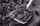20.1 tons of coke is produced from 30 tons of black coal. How much coke is made from 1 kilogram of coal?After the history test, Michaella discovered that the ratio of her correct and incorrect answers is 5: 3. How many correct answers did Michaella have in the test, if she had 6 incorrect answers?
• A cliff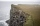A line from the top of a cliff to the ground passes just over the top of a pole 5 ft high and meets the ground at a point 8 ft from the base of the pole. If the point is 93 ft from the base of the​ cliff, how high is the​ cliff?
• Mr. Ben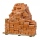Mr. Ben drives bricks to the construction site. If he drove three times a day, he would make bricks in 8 days. How many times a day would he go every day to be done 2 days earlier?
• Everyone drinks the same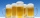24 bricklayers drink 72 beverage bottles a day at the construction site. How many bottles would 19 bricklayers need? Everyone drinks the same.
• Vertical rodThe vertical one meter long rod casts a shadow 150 cm long. Calculate the height of a column whose shadow is 36 m long at the same time.
• The string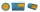They cut 113 cm from the string and divided the rest in a ratio of 5: 6.5: 8: 9.5. The longest part measured 38 cm. Find the original length of the string.
• GearsThe front gear on the bike has 32 teeth and the rear, on the wheel, has 12 teeth. How many times does the rear wheel of the bike turns if you turn the right pedal 30 times? What distance will you go if the circumference of the bicycle wheel is 250 cm?
• Sphere in coneA sphere is inscribed in the cone (the intersection of their boundaries consists of a circle and one point). The ratio of the surface of the ball and the contents of the base is 4: 3. A plane passing through the axis of a cone cuts the cone in an isoscele
• Chimney and treeCalculate the height of the factory chimney, which casts a shadow 6.5 m long in the afternoon. At the same time, a 6 m high tree standing near it casts a shadow 25 dm long.
• Mixing paint with waterMr. Adamek will paint. The purchased paint is diluted with water in a ratio of 1: 1.5. a) how many parts of water will add to 1 part of the paint b) how many liters of water the mission adds to 2 liters of paint
• Equilateral coneWe pour so much water into a container that has the shape of an equilateral cone, the base of which has a radius r = 6 cm, that one-third of the volume of the cone is filled. How high will the water reach if we turn the cone upside down?
• Powerplant chimneyFrom the window of the building at a height of 7.5 m, the top of the factory chimney can be seen at an altitude angle of 76° 30 ′. The base of the chimney can be seen from the same place at a depth angle of 5° 50 ′. How tall is the chimney?